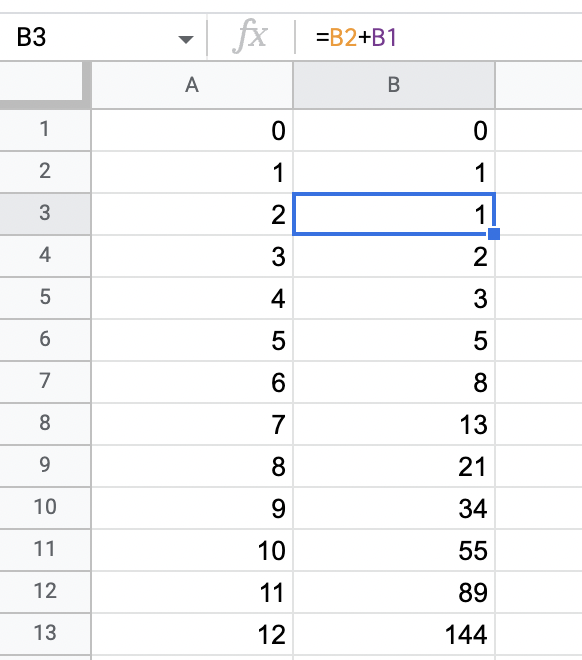This is an article in a series of articles. An overview of the entire project can be found here.

Over the course of this project, small intermedios will occur. Today is one of these. The past days I implemented Fibonacci in what is considered "tradition" programming languages. They have a formal syntax based on a grammar and an alphabet.

Today I am presenting Fibonacci through a tool that many people use on a daily basis. Also, people who do not come out of a computer science / engineering world.

I simply implemented Fibonacci by hard coding two values, 0 and 1, in two cells. These are the values we earlier on has seen as the base cases. Thereafter I used the formula =B2+B1 in the third cell. I could then simply drag and drop that cell allowing the spreadsheet to calculate the respective values.The column left to the Fibonacci column is the index of the corresponding Fibonacci number.

I have written that this method is dynamic programming using tabulation. The reason for this is that I assume this being a pre-calculated table of Fibonacci numbers. When one has this table she can quickly look up the number she needs for further calculation. There might be other classifications of this technique using other reasons.

As usual, the file is in my Fibonacci snippet folder and can be found here.